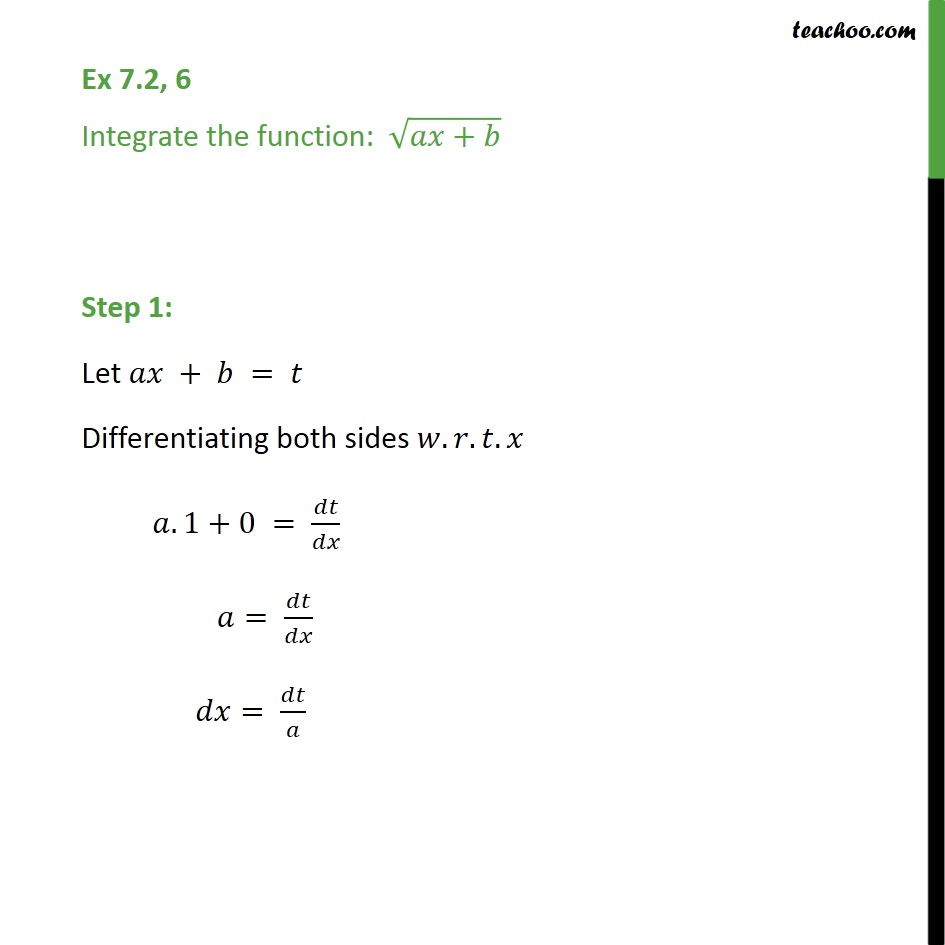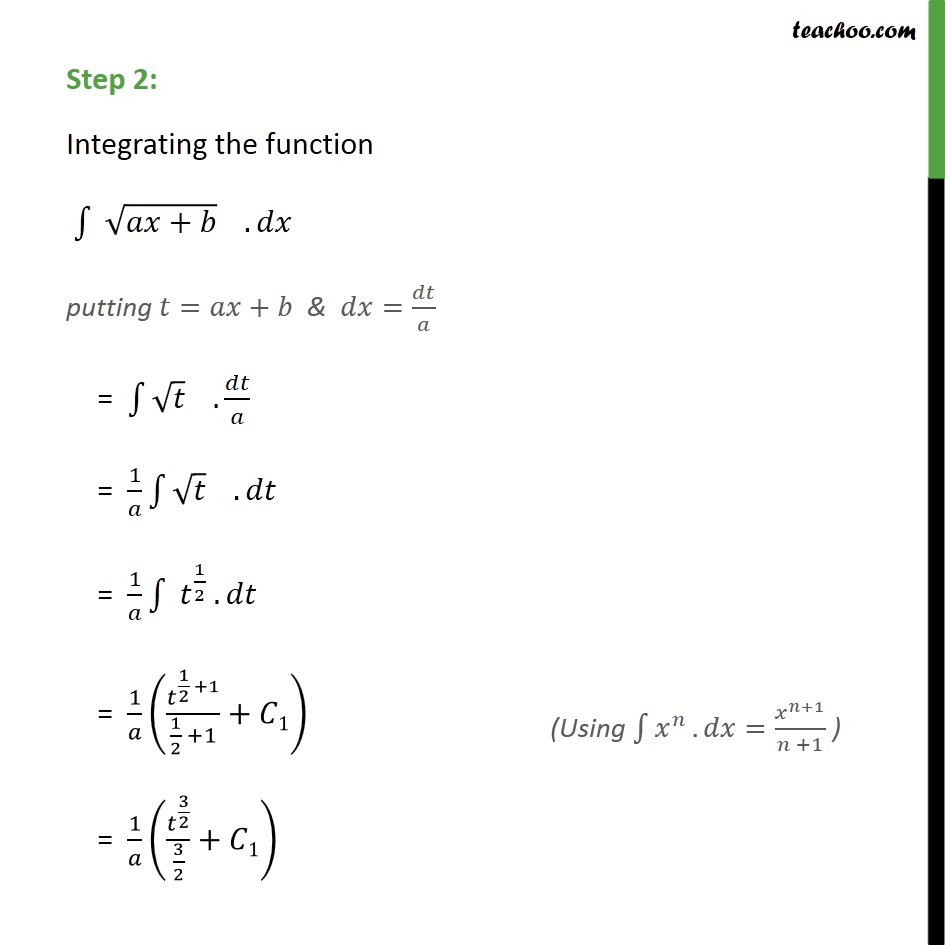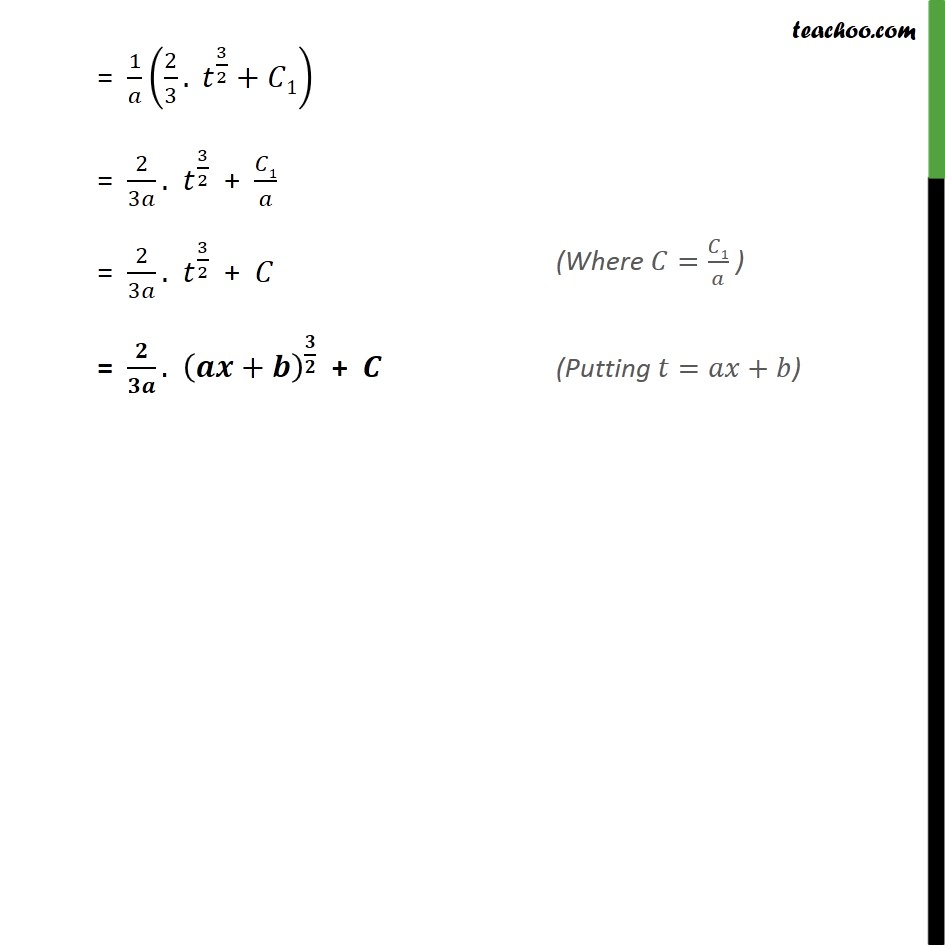Integration by substitution - x^n

Chapter 7 Class 12 Integrals
Concept wiseLearn in your speed, with individual attention - Teachoo Maths 1-on-1 Class

### Transcript

Ex 7.2, 6 Integrate the function: + Step 1: Let + = Differentiating both sides . . . .1+0 = = = Step 2: Integrating the function + . putting = + & = = . = 1 . = 1 1 2 . = 1 1 2 +1 1 2 +1 + 1 = 1 3 2 3 2 + 1 = 1 2 3 . 3 2 + 1 = 2 3 . 3 2 + 1 = 2 3 . 3 2 + = . + +